CBSE Class 12 Sample Paper for 2021 Boards

Class 12
Solutions of Sample Papers and Past Year Papers - for Class 12 Boards

## y + 2z = 7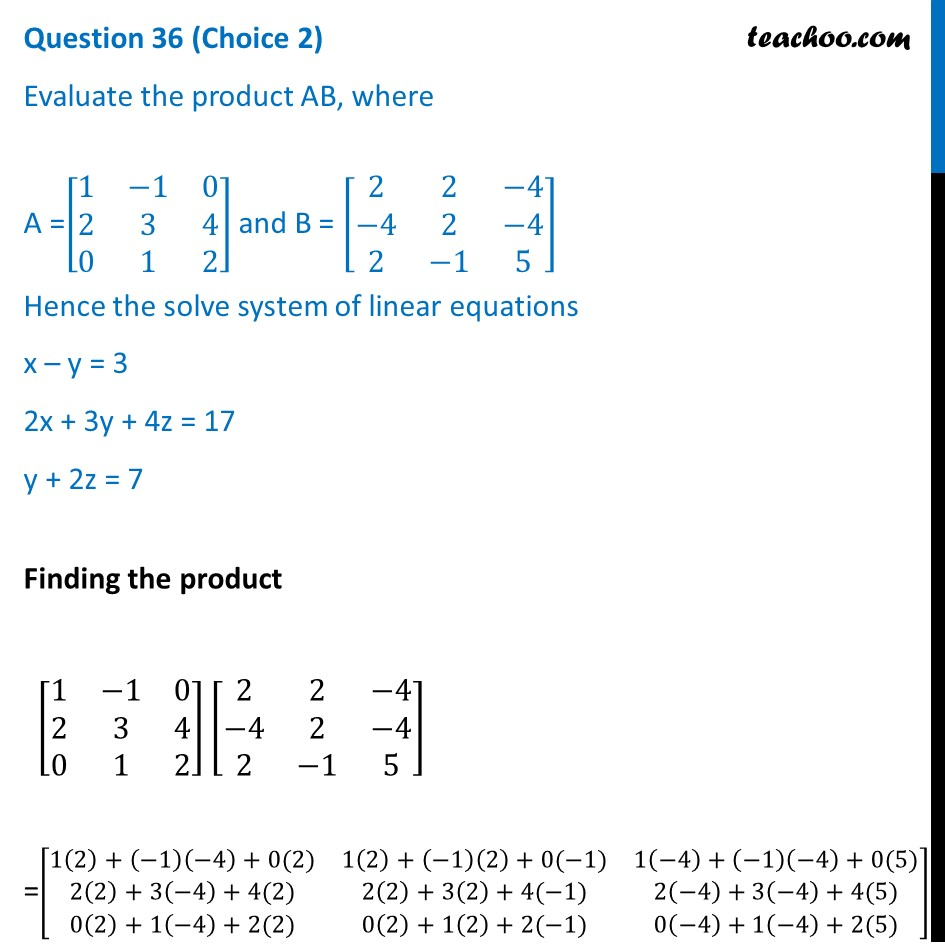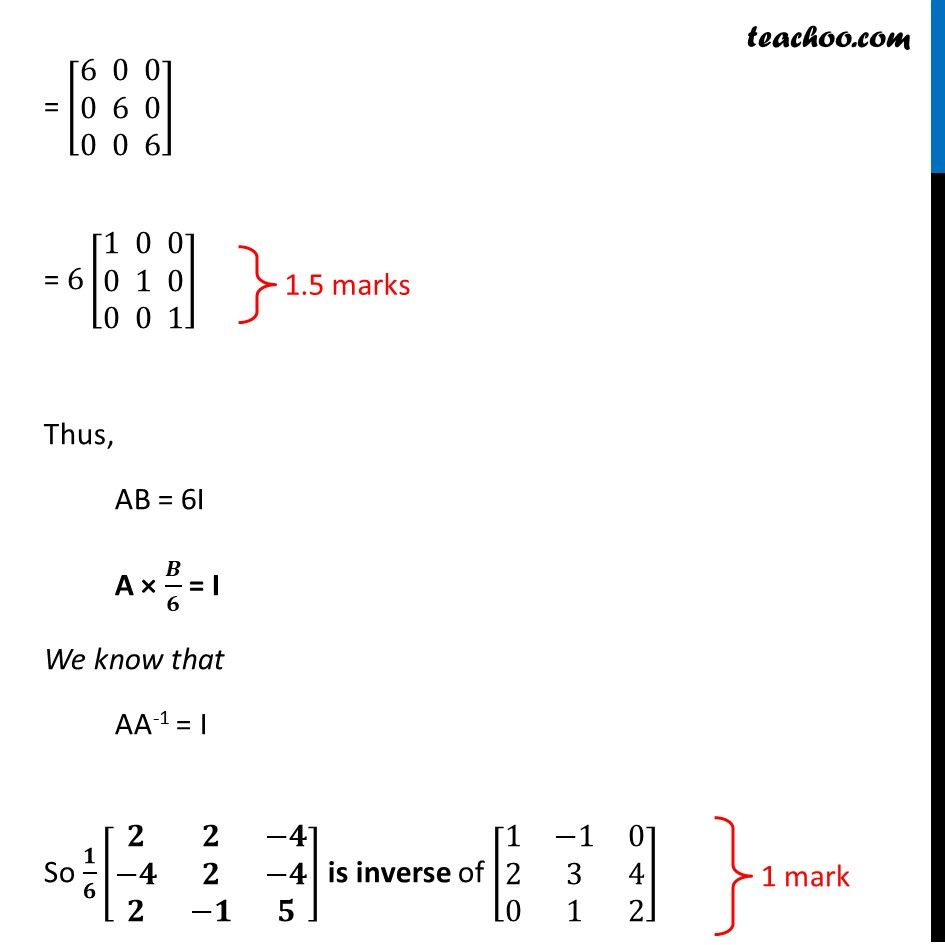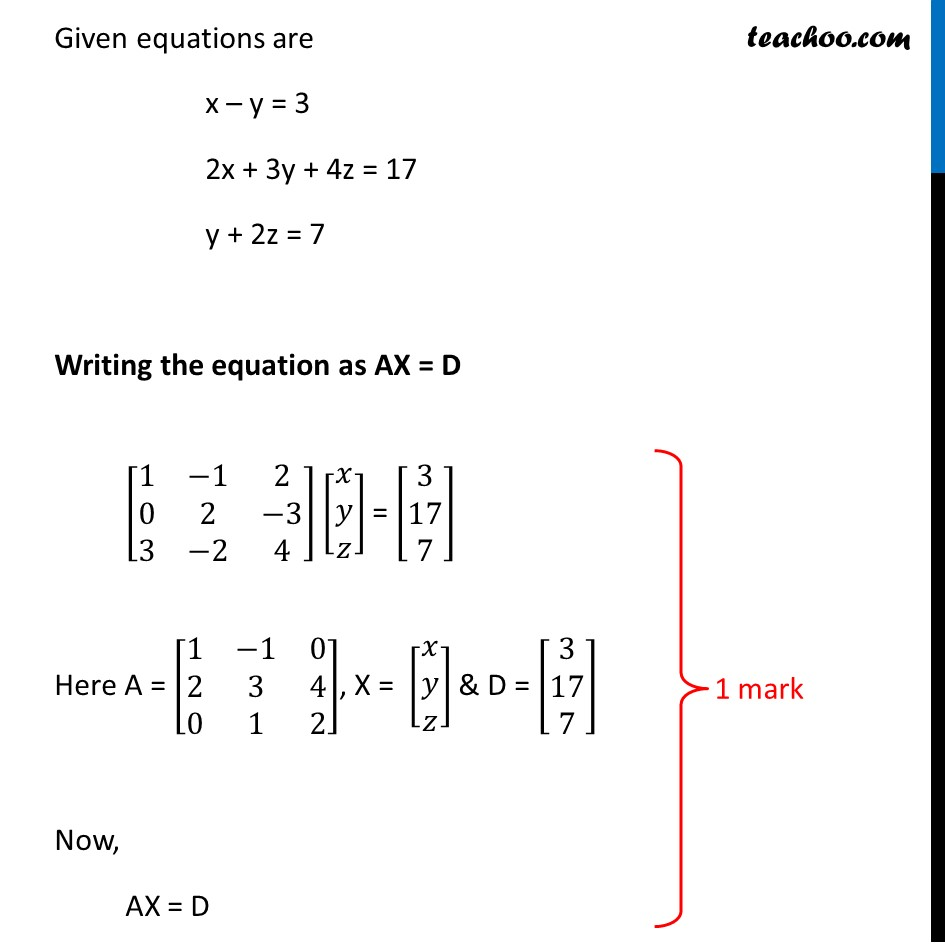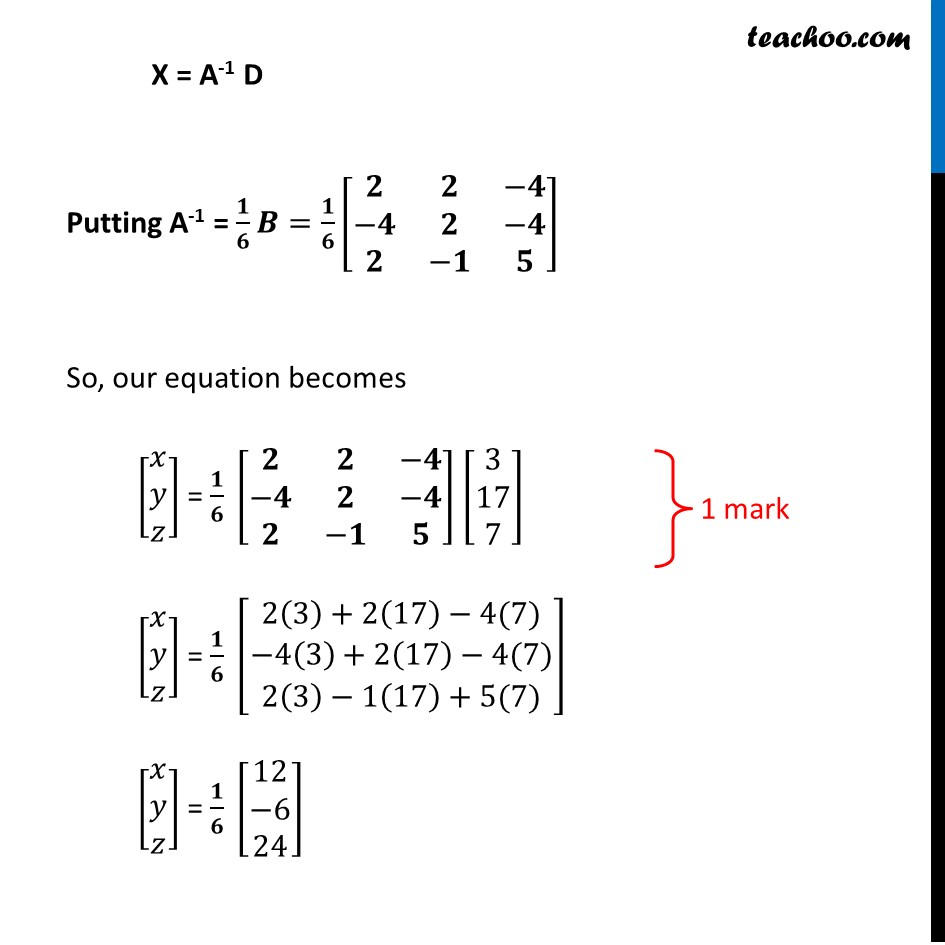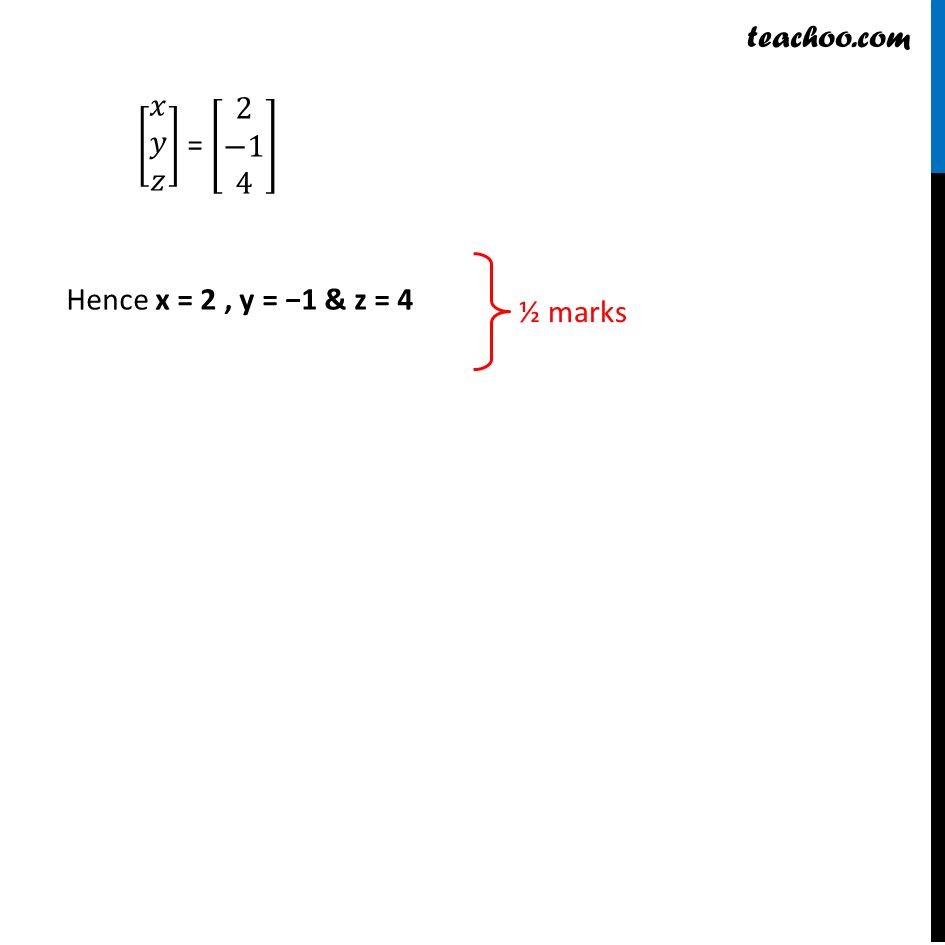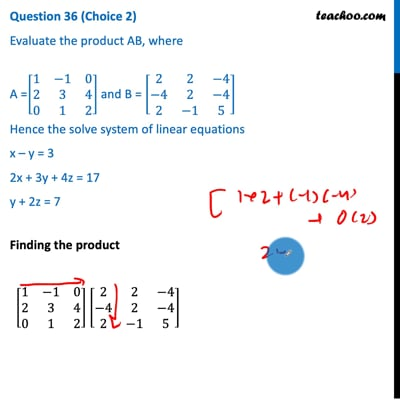This video is only available for Teachoo black users

Note : This is similar to Example 33 of NCERT – Chapter 4 Class 12 Determinants

Introducing your new favourite teacher - Teachoo Black, at only ₹83 per month

### Transcript

Question 36 (Choice 2) Evaluate the product AB, where A =[■8(1&−1&0@2&3&4@0&1&2)] and B = [■8(2&2&−4@−4&2&−4@2&−1&5)] Hence the solve system of linear equations x – y = 3 2x + 3y + 4z = 17 y + 2z = 7 Finding the product [■8(1&−1&0@2&3&4@0&1&2)] [■8(2&2&−4@−4&2&−4@2&−1&5)] =[■8(1(2)+(⤶7−1)(−4)+0(2)&1(2)+(−1)(2)+0(−1)&1(−4)+(−1)(−4)+0(5)@2(2)+3(−4)+4(2)&2(2)+3(2)+4(−1)&2(−4)+3(−4)+4(5)@0(2)+1(−4)+2(2)&0(2)+1(2)+2(−1)&0(−4)+1(−4)+2(5))] = [■8(6@0@0)" " ■8(0@6@0)" " ■8(0@0@6)] = 6[■8(1@0@0)" " ■8(0@1@0)" " ■8(0@0@1)] Thus, AB = 6I A × 𝑩/𝟔 = I We know that AA-1 = I So 𝟏/𝟔 [■8(𝟐&𝟐&−𝟒@−𝟒&𝟐&−𝟒@𝟐&−𝟏&𝟓)] is inverse of [■8(1&−1&0@2&3&4@0&1&2)] Given equations are x – y = 3 2x + 3y + 4z = 17 y + 2z = 7 Writing the equation as AX = D [■8(1&−1&2@0&2&−3@3&−2&4)] [■8(𝑥@𝑦@𝑧)] = [■8(3@17@7)] Here A = [■8(1&−1&0@2&3&4@0&1&2)], X = [■8(𝑥@𝑦@𝑧)] & D = [■8(3@17@7)] Now, AX = D X = A-1 D Putting A-1 = 𝟏/𝟔 𝑩=𝟏/𝟔 [■8(𝟐&𝟐&−𝟒@−𝟒&𝟐&−𝟒@𝟐&−𝟏&𝟓)] So, our equation becomes [■8(𝑥@𝑦@𝑧)] = 𝟏/𝟔 [■8(𝟐&𝟐&−𝟒@−𝟒&𝟐&−𝟒@𝟐&−𝟏&𝟓)] [■8(3@17@7)] [■8(𝑥@𝑦@𝑧)] = 𝟏/𝟔 [■8(2(3)+2(17)−4(7)@−4(3)+2(17)−4(7)@2(3)−1(17)+5(7))] [■8(𝑥@𝑦@𝑧)] = 𝟏/𝟔 [■8(12@−6@24)] [■8(𝑥@𝑦@𝑧)] = [■8(2@−1@4)] Hence x = 2 , y = −1 & z = 4